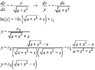# ? solution of ( dy / dx ) + ( y / (sqrt(a+(x^2))) ) = 0

• smslca
In summary, the given differential equation is solved by using a trig substitution to find the indefinite integral, which is then used to find the general solution. The only solution to the equation is y = √(a + x2) - x, and there are no other solutions.

#### smslca

If y = f(x) , and ( dy / dx ) + ( y / ( sqrt(a+(x^2)) ) ) = 0

I knew its solution is y = { sqrt(a+(x^2)) - x } , where a is a constant

can anyone give the proof , by solving the differntial equation.

Are there any other solutions for the above given differential equation. I asked this other
solutions because, on rearranging the given differential equation we get

( dy / y ) = - ( dx / ( sqrt(a+(x^2)) ) )
on integrating
ln y = { integral ( - ( dx / ( sqrt(a+(x^2)) ) ) ) } + ln c

so I may get the solution as y = ce^y1 , where y1 is funcion of x other than f(x)

I am not sure about the existence of general solution , but I think it may exist.

_____________________________________________________

so what I want is ,

1. Solving procedure for the differntial equation to get the solution
y = { sqrt(a+(x^2)) - x }

2. what is general answer for { integral ( - ( dx / ( sqrt(a+(x^2)) ) ) }

3. Is there any general solution for the differential equation given.
other than y = { sqrt(a+(x^2)) - x }

______________________________________________________

Procedures I have tried and falied to do further.

1. we know d(sqrt(a+(x^2))) / dx = x / sqrt(a+(x^2))
so 1 / sqrt(a+(x^2)) = ( d(sqrt(a+(x^2))) / dx ) /x
on substituting this value in the differential equation , we will get
( dy / dx ) + ( ( y * d( sqrt(a+(x^2)))/dx ) / x ) = 0
on solving this I got strucked at
ln y = ( - ( sqrt(a+(x^2)) ) / x ) - { integral ( sqrt(a+(x^2)) / x^2) dx )

2. rearranging the differntial equation we get
( dy / y ) = - ( dx / ( sqrt(a+(x^2)) ) )
take x = a cos(t)
dx = - a sin(t) dt
t = cos^-1 (x/a)

on solving I got strucked at
( dy / y ) = { ( sqrt(2p) * sin(t) ) / sqrt( cos(2t) + 3 ) } dt

I got no other ideas. I wish , I can get the answers for all the 3 questions I have asked.

hi smslca!(have an integral: ∫ and a square-root: √ and try using the X2 icon just above the Reply box)
smslca said:
If y = f(x) , and ( dy / dx ) + ( y / ( sqrt(a+(x^2)) ) ) = 0

I knew its solution is y = { sqrt(a+(x^2)) - x } , where a is a constant

can anyone give the proof , by solving the differntial equation.

Are there any other solutions for the above given differential equation. I asked this other
solutions because, on rearranging the given differential equation we get

( dy / y ) = - ( dx / ( sqrt(a+(x^2)) ) )
on integrating
ln y = { integral ( - ( dx / ( sqrt(a+(x^2)) ) ) ) } + ln c

no

∫ dx/√(a + x2) dx is not √(a + x2) - x + Cuse a trig substitution, and try againtiny-tim said:
no

∫ dx/√(a + x2) dx is not √(a + x2) - x + C

I said y = √(a + x2) - x is the solution for ( dy / dx ) + ( y / ( sqrt(a+(x^2)) ) ) = 0

not for ∫ dx/√(a + x2)

smslca said:
I said y = √(a + x2) - x is the solution for ( dy / dx ) + ( y / ( sqrt(a+(x^2)) ) ) = 0

not for ∫ dx/√(a + x2)

but if ( dy / dx ) + ( y / ( sqrt(a+(x^2)) ) ) = 0

then dy/y = -dx/√(a + x2),

and ln y = -∫ dx/√(a + x2) + CHave you tried plugging in the supposed solution and working through the algebra?

I said y = √(a + (x^2)) - x is the solution for ( dy / dx ) + ( y / ( sqrt(a+(x^2)) ) ) = 0
You said wrong because y = √(a + (x^2)) - x is NOT a solution for ( dy / dx ) + ( y / ( sqrt(a+(x^2)) ) ) = 0

JJacquelin said:
You said wrong because y = √(a + (x^2)) - x is NOT a solution for ( dy / dx ) + ( y / ( sqrt(a+(x^2)) ) ) = 0

It isn't?

$$\frac{dy}{dx}+\frac{y}{\sqrt{a+x^{2}}}=0$$

$$y=\sqrt{a+x^{2}}-x$$

$$\frac{d(\sqrt{a+x^{2}}-x)}{dx}+\frac{\sqrt{a+x^{2}}-x}{\sqrt{a+x^{2}}}=0$$

$$\frac{1}{2\sqrt{a+x^{2}}}\frac{d(a+x^{2})}{dx}-1+\frac{\sqrt{a+x^{2}}-x}{\sqrt{a+x^{2}}}=0$$

$$\frac{1}{2\sqrt{a+x^{2}}}\cdot2x-1+\frac{\sqrt{a+x^{2}}-x}{\sqrt{a+x^{2}}}=0$$

$$\frac{2x}{2\sqrt{a+x^{2}}}-1+\frac{\sqrt{a+x^{2}}}{\sqrt{a+x^{2}}}-\frac{x}{\sqrt{a+x^{2}}}=0$$

$$\frac{x}{\sqrt{a+x^{2}}}-1+1-\frac{x}{\sqrt{a+x^{2}}}=0$$

$$\frac{x}{\sqrt{a+x^{2}}}-\frac{x}{\sqrt{a+x^{2}}}-1+1=0$$

$$0=0$$

Sorry, excuse me for my mistake

#### Attachments

•EDO.JPG
10.5 KB · Views: 1,031
quicker is dy/y = -∫ dx/√(a + x2) dx

so lny = - ln|x + √(a + x2)| + aC (from the standard integrals list)

(or go straight to ln|x - √(a + x2)| if you happen to know it!)

so y = aC/(x + √(a + x2)) = aC(√(a + x2) - x)/((a + x2) - x2)

= C(√(a + x2) - x)